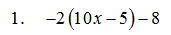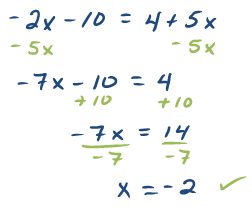## Algebra

Showing posts with label like terms. Show all posts
Showing posts with label like terms. Show all posts

### Elementary Algebra Exam #1

Click on the 10 question exam covering topics in chapters 1 and 2. Give yourself one hour to try all of the problems and then come back and check your answers.

Simplify:Solve:Solve and graph all solutions on a number line.10. The perimeter of a rectangle is 54 feet.  If the length is 3 feet less than twice the width, find the dimensions of the rectangle. (Set up an algebraic equation and use it to solve this problem.)